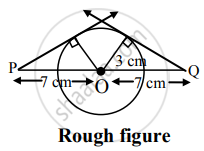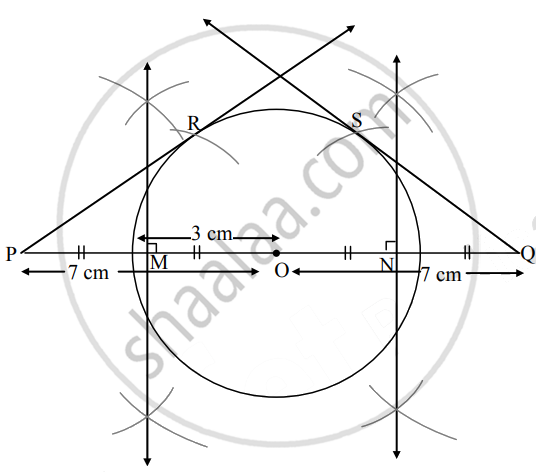# Draw a circle with center O and radius 3 cm. Take the point P and the point Q at a distance of 7 cm from the center of the circle on the opposit - Geometry

Diagram

Draw a circle with center O and radius 3 cm. Take the point P and the point Q at a distance of 7 cm from the center of the circle on the opposite side of the circle such that their line of intersection passing through the center of the circle Draw a tangent to the circle from the point P and the point Q

#### SolutionSteps of construction:

1. With centre O, draw a circle of radius 3 cm.
2. Take point P and Q such that OP = 7 cm and OQ = 7 cm.
3. Draw the perpendicular bisector of seg OP. It intersects OP in point M.
4. Also, draw the perpendicular bisector of seg OQ. It intersects OQ in point N.
5. With M as centre and radius equal to PM, draw an arc intersecting the circle in points R and with N as centre and radius equal to NQ, draw an arc intersecting the circle in points S.
6. Draw rays PR and QS.
Rays PR and QS are the required tangents to the circle.
Concept: Construction of a Tangent to the Circle at a Point on the Circle
Is there an error in this question or solution?

Share